#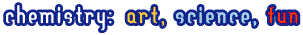### Moscow-1996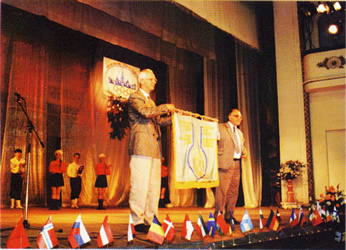1996 International Jury session 1996 Russia passes the flag to Canada

##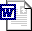Theoretical Problem 3

In 1908 E.Rutherford together with H.Geiger measured the rate ( x ) of emission of a -particles by radium (in the nature this element is represented by a single nuclide 226 Ra) and found that 1.00 g of radium emits x = 3.42 * 10 10 a -particles per second.

In 1911 Rutherford and American physical chemist B.Boltwood measured the rate of formation of helium from radium. This experiment permitted to obtain the most accurate value of Avogadro's number available at that time, given that the value of molar volume of ideal gas was well established. To achieve this goal a sample of radium salt purified from decay products and containing m = 192 mg of Ra was put into a device and the volume of the evolved helium was measured.

After 83 days ( t = 83.0 days) of the experiment 6.58 mm 3 of He was collected ( V He = 6.58 mm 3 corrected to 0 o C and 1 atm). To understand the results of this experiment we shall need the kinetic scheme of radioactive decay of Ra which is given below (half-lives are over the arrow, the type of decay is below the arrows):(RaA - RaE are intermediate products of radon decay).

1. Write down the first six radioactive decays using a modern notation showing atomic and mass numbers of all nuclei involved.

In a rough approximation, half-lives of all radium decay products, except those of RaD and Po, may be assumed to be negligible compared to the time of measurement, t . Using this approximation perform the following calculations:

2. a) How many helium atoms were formed from each decayed radium atom after 83 days"

b) How many helium atoms were formed in total during the experiment"

3. Calculate an approximate value of Avogadro's number from the above data.

For a more accurate computation of Avogadro's number the half-life of radon T 1/2 (Rn) = 3.83 days cannot be neglected as it is comparable with the duration of experiment t and not all of radon atoms decayed to the end of experiment.

4. Choose which of the plots given below displays the time dependence of the number of radon atoms N Rn in the course of the experiment.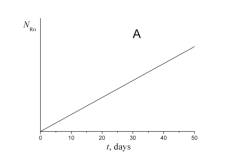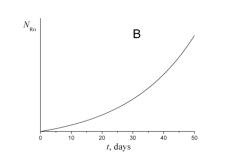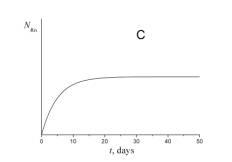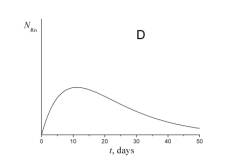5. Choose which of the plots given below shows the time dependence of the volume of helium V He of radon atoms in the course of the experiment.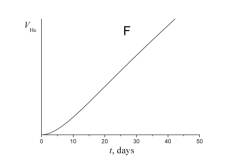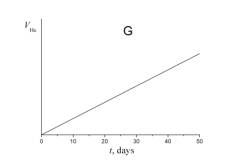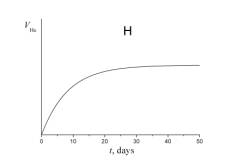6. a) Using a short kinetic scheme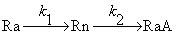and the plot which you have selected in question 4, write a relation between the number of radon atoms at the end of experiment N ¢ Rn and the number of radium atoms N Ra .

b) Evaluate N ¢ Rn using the rate of radium decay given above ( x = 3.42 * 10 10 a -particles per gram of radium per second).

7. How many helium atoms could be formed from radon atoms remaining at the end of experiment N ¢ Rn if all these atoms had decayed to RaD"

8. Using your answers to the above questions calculate a better approximation to: a) the number of helium atoms formed; b) the Avogadro's number.

##Solution

______________________________________________________________________

##Theoretical Problem 4

Inorganic chemistry

Potassium dichromate is one of the most widely used precipitating reagents. The following equilibria are established in aqueous solutions of Cr(VI).
HCrO4- + H2O = CrO42- + H3O+ pK1 = 6.50
2HCrO4- = Cr2O72- + H2O pK2 = -1.36
All other equlibria with involving of chromium should be ignored. All the activity coefficients are assumed to be 1.
1. The ionic product of water Kw = 1.0∙10-14. Evaluate the equilibrium constants
a) CrO42- + H2O = HCrO4- + OH-
b) Cr2O72- + 2OH- = 2CrO42- + H2O
2. The solubility product of BaCrO4 Ks = 1.2∙10-10. BaCr2O7 is very soluble in water. In what direction will the equilibrium 1b shift upon the addition of the following reagents to a moderately concentrated aqueous solution of potassium dichromate?
a) KOH
b) HCl
c) BaCl2
d) H2O (consider all the above equilibria).
3. Dissociation constant of acetic acid Ka = 1.8∙10-5. Calculate the pH value of the following solutions
a) 0.010 M K2CrO4
b) 0.010 M K2Cr2O7
c) 0.010 M K2Cr2O7 + 0.10 M CH3COOH
4. Calculate the equilibrium concentrations of the following ions in a solution of 0.010 M K2Cr2O7 + 0.10 M CH3COOH
a) CrO42-
b) Cr2O72-

##Solution

______________________________________________________________________

##Theoretical Problem 7

Organic chemistry

Stereochemistry of organic compounds can sometimes be determined by studying their chemical behavior. The stereochemical configuration of one of the isomers of 5 nonbornene 2,3 dicarboxilyc acids (compound X)(no stereochemistry is shown) was established by the following experiments.

On heating this substance decomposes producing water and a new compound Y. Compound Y slowly dissolves in excess of aqueous NaOH with the formation of product X1 same to that is formed in the reaction of X with NaOH. The resulting solution of X1 is treated by I2 to give compounds containing iodine. Acidification of the solution leads to a mixture of two isomeric compounds, A and B in the 3:1 ratio. The titration of 0.3913 g of compound A by 0.1000 M aqueous solution of NaOH in the presence of phenolphthalein takes 12.70 ml of alkali. The same amount of 0.1000 M solution of NaOH is required for the titration of 0.3913 g of compound B. On heating compound A slowly transforms into compound C, which contains no iodine and is able to react with water. Under the same conditions compound B does no undergo this transformation, but on heating with hydrochloric acid slowly transforms into A.

All reactions must be written as balance equations. No mechanisms is required.

1. Mark by asterisks (*) the asymmetric carbon atoms in the structure of 5 nonbornene 2,3 dicarboxilyc acids.

2. Draw the stereochemical formulas of all stereoisomers of compound X, and the structures of products of their dehydration in those cases when it is possible.

3. Write the reactions of NaOH with a stereoisomer of X and a stereoisomer of Y.

4. Calculate the molecular mass of compound A. Write the reactions leading from X1 to A.

5. Write the reaction of the formation of C from A and the reaction of C with water.

6. Draw the stereochemical formula of compound X, which satisfies all of the data given in the problem.

7. Write the reactions leading from B to A.

8. Are the compounds A and B diastereomers?

##Solution

| ©2006-2007 Chemisty Department of Moscow State University |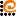© web-design www.AdCode.ru